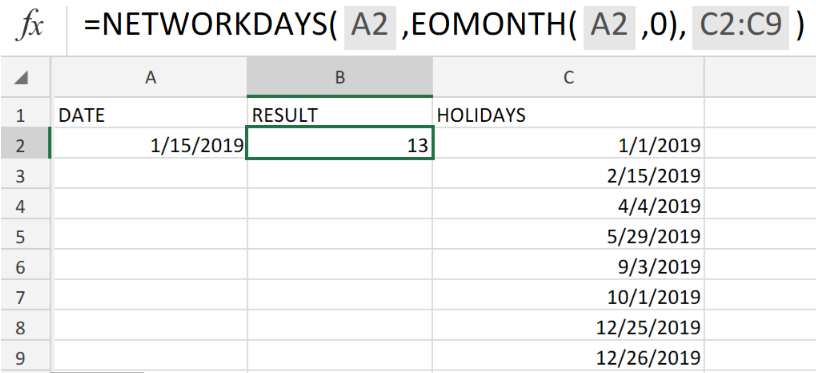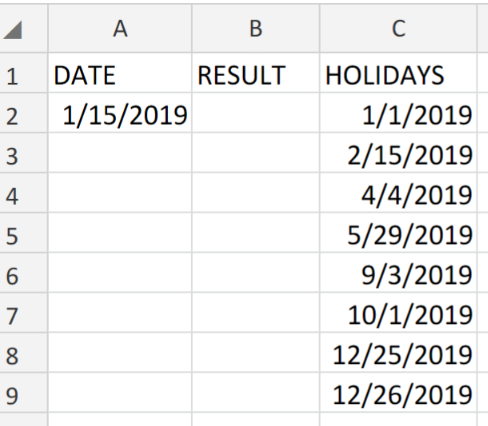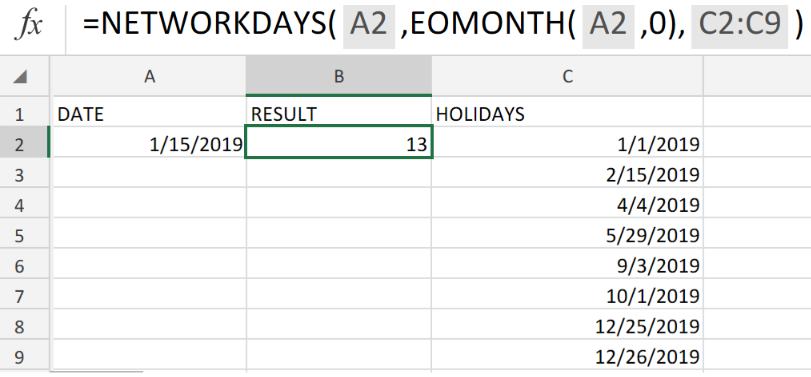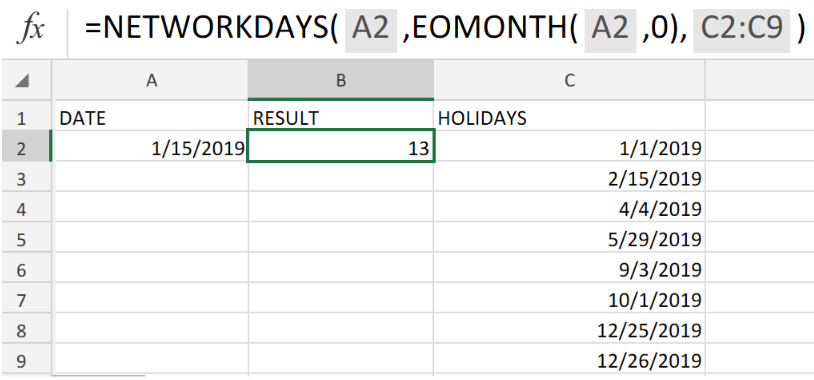Get instant live expert help with Excel or Google Sheets“My Excelchat expert helped me in less than 20 minutes, saving me what would have been 5 hours of work!”

#### Post your problem and you’ll get expert help in seconds.

Your message must be at least 40 characters
Our professional experts are available now. Your privacy is guaranteed.

# Working days left in monthFigure 1. Working Days Left in Month

We are going to demonstrate an effective method of determining the actual number of Working Days Left in a Month.

To do this, we will be using the Excel NETWORKDAYS function.

## Generic Formula

`=NETWORKDAYS(B5,EOMONTH(B5,0),E5:E14)`

This inbuilt Excel Function requires a specified start date, end date, as well as a range of holiday dates.

## How to use the Excel NETWORKDAYS function.

To use the NETWORKDAYS function to calculate the actual number of working days left in a month, we are going to follow 3 simple steps;

1. Gather all the information available to us and arrange them in our worksheet. Make sure to provide a blank cell for Excel to determine the remaining number of working days.

See example illustrated below;Figure 2. Working Days Left in Month Excel.

Our purpose here is to calculate the total amount of monthly working days left within the specified range of dates (public holidays excluded).

1. Enter a custom version of the NETWORKDAYS formula into the formula bar for cell B2 of our worksheet to get the desired result.

The modified formula for cell B2 is as follows;

`=NETWORKDAYS(A2,EOMONTH(A2,0),C2:C9)`Figure 3. Working Days Left in Month Excel.

Where cell A2 contains our specified current date, and the range of cells, C2:C9 contains specific holiday dates.

## Note

both the start and end dates must be included in the NETWORKDAYS calculation, if they are actual working days.

In the example illustrated above, our start date is Jan 15, 2019, provided in cell A2. Our end date is determined with the EOMONTH function using an offset value of 0, which will automatically return the last day of the month of the specified date. Our list of holiday dates is contained within the cell range C2:C9.Figure 4. Working Days Left in Month.

## Instant Connection to an Expert through our Excelchat Service:

Our live Excelchat Service is here for you. We have Excel Experts available 24/7 to answer any Excel questions you may have. Guaranteed connection within 30 seconds and a customized solution for you within 20 minutes.

### Did this post not answer your question? Get a solution from connecting with the expert.Another blog reader asked this question today on Excelchat:
Solution examplesI need to sum the number of hours per month one of three halls are being rented
Solved by A. F. in 60 minsneed help with the different sheets and formula IF(A4=B4,0,NETWORKDAYS(A4+1,B4,\$E\$9:\$E\$42) +0.5*(INT((+B4-A4+1)/7)+IF(WEEKDAY(A4+1)+MOD (B4-A4-1,7)>=7,1,0)))
Solved by O. D. in 11 minsi have this formula currently which works but not if I want it for every day - any ideas ?? (NETWORKDAYS.INTL(L1362,M1362,11,BH!A:A)-1)*("17:30"-"8:30")+IF(NETWORKDAYS.INTL(M1362,M1362,11,BH!A:A),MEDIAN(MOD(M1362,1),"8:30","17:30"),"17:30")-MEDIAN(NETWORKDAYS.INTL(L1362,L1362,11,BH!A:A)*MOD(L1362,1),"8:30","17:30")
Solved by A. D. in 28 mins## Subscribe to Excelchat.coAnother blog reader asked this question today on Excelchat: